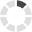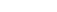### Site InformationLoading... Please wait...

# MATH302 Midterm Exam (APUS)

\$25.00

## Product Description

MATH302 Midterm Exam (APUS)

If a variable has possible values –2, 6, and 17, then this variable is

If two events are independent, what is the probability that they both occur?

What is the probability of drawing two queens in a row from a standard deck of cards without

The following data were obtained from a survey of college students. The variable X represents the number of non-assigned books read during the past six months.

Suppose that 50 identical batteries are being tested. After 8 hours of continuous use, assume that a given battery is still operating with a probability of 0.70 and has failed with a probability of 0.30.

Suppose that 50 identical batteries are being tested. After 8 hours of continuous use, assume that a given battery is still operating with a probability of 0.70 and has failed with a probability of 0.30.

What is the probability that fewer than 40 batteries will last at least 8 hours?

The mean of a probability distribution can be:

The standard deviation of is usually called the

If the value of the standard normal random variable Z is positive, then the original score is where in relationship to the mean?

The average height of flowering cherry trees in a nursery is 11 feet. If the heights are normally distributed with a standard deviation of 1.6, find the probability that a randomly

selected cherry tree in this nursery is less than 13 feet tall.

The continuous distribution characterized by a symmetric, bell-shaped curve is the:

One reason for standardizing random variables is to measure variables with:

Accepted characters: numbers, decimal point markers (period or comma), sign indicators (-), spaces (e.g., as thousands separator, 5 000), "E" or "e" (used in scientific notation). NOTE: For scientific notation, a period MUST be used as the decimal point marker.
Complex numbers should be in the form (a + bi) where "a" and "b" need to have explicitly stated values. For example: {1+1i} is valid whereas {1+i} is not. {0+9i} is valid whereas {9i} is not.

The histogram below represents scores achieved by 250 job applicants on a personality profile.

Accepted characters: numbers, decimal point markers (period or comma), sign indicators (-), spaces (e.g., as thousands separator, 5 000), "E" or "e" (used in scientific notation). NOTE: For scientific notation, a period MUST be used as the decimal point marker.
Complex numbers should be in the form (a + bi) where "a" and "b" need to have explicitly stated values. For example: {1+1i} is valid whereas {1+i} is not. {0+9i} is valid whereas {9i} is not.

In February 2002 the Argentine peso lost 70% of its value compared to the United States dollar. This devaluation drastically raised the price of imported products. According to a survey conducted by AC Nielsen in April 2002, 68% of the consumers in Argentina were buying fewer products than before the devaluation, 24% were buying the same number of products, and 8% were buying more

products. Furthermore, in a trend toward purchasing less-expensive brands, 88% indicated that they had changed the brands they purchased. Suppose the following complete set of results were reported. Use the following data to answer this question.

Accepted characters: numbers, decimal point markers (period or comma), sign indicators (-), spaces (e.g., as thousands separator, 5 000), "E" or "e" (used in scientific notation). NOTE: For scientific notation, a period MUST be used as the decimal point marker.
Complex numbers should be in the form (a + bi) where "a" and "b" need to have explicitly stated values. For example: {1+1i} is valid whereas {1+i} is not. {0+9i} is valid whereas {9i} is not.

Accepted characters: numbers, decimal point markers (period or comma), sign indicators (-), spaces (e.g., as thousands separator, 5 000), "E" or "e" (used in scientific notation). NOTE: For scientific notation, a period MUST be used as the decimal point marker.
Complex numbers should be in the form (a + bi) where "a" and "b" need to have explicitly stated values. For example: {1+1i} is valid whereas {1+i} is not. {0+9i} is valid whereas {9i} is not.

An ice cream vendor sells three flavors: chocolate, strawberry, and vanilla. Forty five percent of the sales are chocolate, while 30% are strawberry, with the rest vanilla flavored. Sales are by the cone or the cup. The percentages of cones sales for chocolate, strawberry, and vanilla, are 75%, 60%, and 40%, respectively. For a randomly selected sale, define the following events:

Accepted characters: numbers, decimal point markers (period or comma), sign indicators (-), spaces (e.g., as thousands separator, 5 000), "E" or "e" (used in scientific notation). NOTE: For scientific notation, a period MUST be used as the decimal point marker.
Complex numbers should be in the form (a + bi) where "a" and "b" need to have explicitly stated values. For example: {1+1i} is valid whereas {1+i} is not. {0+9i} is valid whereas {9i} is not.

Suppose that a marketing firm sends questionnaires to two different companies. Based on historical evidence, the marketing research firm believes that each company, independently of the other, will return the questionnaire with a probability of 0.30. What is the probability that only one of the questionnaires will be returned? Place your answer, rounded to 2 decimal places, in the blank. For example, 0.23 is a

Accepted characters: numbers, decimal point markers (period or comma), sign indicators (-), spaces (e.g., as thousands separator, 5 000), "E" or "e" (used in scientific notation). NOTE: For scientific notation, a period MUST be used as the decimal point marker.
Complex numbers should be in the form (a + bi) where "a" and "b" need to have explicitly stated values. For example: {1+1i} is valid whereas {1+i} is not. {0+9i} is valid whereas {9i} is not.

Accepted characters: numbers, decimal point markers (period or comma), sign indicators (-), spaces (e.g., as thousands separator, 5 000), "E" or "e" (used in scientific notation). NOTE: For scientific notation, a period MUST be used as the decimal point marker.
Complex numbers should be in the form (a + bi) where "a" and "b" need to have explicitly stated values. For example: {1+1i} is valid whereas {1+i} is not. {0+9i} is valid whereas {9i} is not.

Accepted characters: numbers, decimal point markers (period or comma), sign indicators (-), spaces (e.g., as thousands separator, 5 000), "E" or "e" (used in scientific notation). NOTE: For scientific notation, a period MUST be used as the decimal point marker.
Complex numbers should be in the form (a + bi) where "a" and "b" need to have explicitly stated values. For example: {1+1i} is valid whereas {1+i} is not. {0+9i} is valid whereas {9i} is not.

The mean weight of loads of coal placed in train cars by a loading machine is 43.0 tons with a standard deviation of 8.0 tons. Assuming that the weight of loads placed in the train cars by this loader are normally distributed, if a random sample of 9 loads is chosen for a weight check, find the probability that the mean weight of those loads is more than 40.60 tons.

Accepted characters: numbers, decimal point markers (period or comma), sign indicators (-), spaces (e.g., as thousands separator, 5 000), "E" or "e" (used in scientific notation). NOTE: For scientific notation, a period MUST be used as the decimal point marker.
Complex numbers should be in the form (a + bi) where "a" and "b" need to have explicitly stated values. For example: {1+1i} is valid whereas {1+i} is not. {0+9i} is valid whereas {9i} is not.

Accepted characters: numbers, decimal point markers (period or comma), sign indicators (-), spaces (e.g., as thousands separator, 5 000), "E" or "e" (used in scientific notation). NOTE: For scientific notation, a period MUST be used as the decimal point marker.
Complex numbers should be in the form (a + bi) where "a" and "b" need to have explicitly stated values.

Accepted characters: numbers, decimal point markers (period or comma), sign indicators (-), spaces (e.g., as thousands separator, 5 000), "E" or "e" (used in scientific notation). NOTE: For scientific notation, a period MUST be used as the decimal point marker.
Complex numbers should be in the form (a + bi) where "a" and "b" need to have explicitly stated values. For example: {1+1i} is valid whereas {1+i} is not. {0+9i} is valid whereas {9i} is not.

Using the standard normal curve, the Z- score representing the 75th percentile is approximately 0.674.

A random variable X is standardized when each value of X has the mean of X subtracted from it, and the difference is divided by the standard deviation of X.

## Customers also viewed

• \$25.00• \$25.00• \$25.00• \$25.00• \$25.00## Related Products

• BUSN100 Midterm Exam (APUS) \$25.00• LSTD302 Midterm Exam (APUS) \$25.00• MATH302 Final Exam (APUS) \$25.00• POLS210 Midterm Exam (APUS) \$25.00• SCIN136 Midterm Exam (APUS) \$25.00Click the button below to add the MATH302 Midterm Exam (APUS) to your wish list.